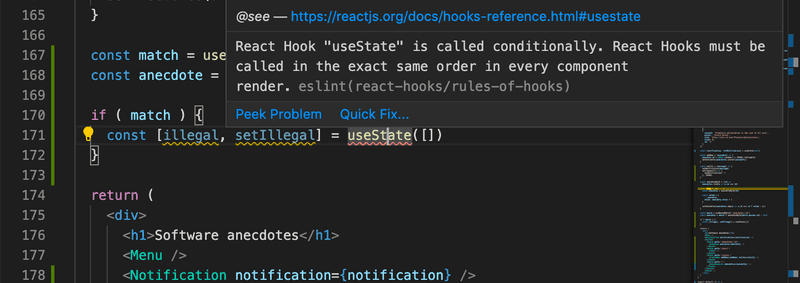b

# 自定义 hooks

### Hooks

React 提供了10种不同的内置Hook，其中最受欢迎的是我们已经广泛使用的useStateuseEffect Hook。

Don’t call Hooks inside loops, conditions, or nested functions. Instead, always use Hooks at the top level of your React function.

Don’t call Hooks from regular JavaScript functions. Instead, you can:

• Call Hooks from React function components.
• 从 React 函数组件调用Hook。
• Call Hooks from custom Hooks
• 从自定义Hook调用Hook

Create-react-app 已经配置好了规则eslint-plugin-react-hooks ，如果Hook被非法使用就会产生警告:### Custom hooks

【自定义Hook】

React 提供了创建我们自己的自定义Hook的选项。 根据 React，自定义Hook的主要目的是促进组件中使用的逻辑的重用。

``````import React, { useState } from 'react'
const App = (props) => {
const [counter, setCounter] = useState(0)

return (
<div>
<div>{counter}</div>
<button onClick={() => setCounter(counter + 1)}>
plus
</button>
<button onClick={() => setCounter(counter - 1)}>
minus
</button>
<button onClick={() => setCounter(0)}>
zero
</button>
</div>
)
}``````

``````const useCounter = () => {
const [value, setValue] = useState(0)

const increase = () => {
setValue(value + 1)
}

const decrease = () => {
setValue(value - 1)
}

const zero = () => {
setValue(0)
}

return {
value,
increase,
decrease,
zero
}
}``````

React组件可以使用如下所示的Hook:

``````const App = (props) => {
const counter = useCounter()

return (
<div>
<div>{counter.value}</div>
<button onClick={counter.increase}>
plus
</button>
<button onClick={counter.decrease}>
minus
</button>
<button onClick={counter.zero}>
zero
</button>
</div>
)
}``````

``````const App = () => {
const left = useCounter()
const right = useCounter()

return (
<div>
{left.value}
<button onClick={left.increase}>
left
</button>
<button onClick={right.increase}>
right
</button>
{right.value}
</div>
)
}``````

``````const App = () => {
const [name, setName] = useState('')
const [born, setBorn] = useState('')
const [height, setHeight] = useState('')

return (
<div>
<form>
name:
<input
type='text'
value={name}
onChange={(event) => setName(event.target.value)}
/>
<br/>
birthdate:
<input
type='date'
value={born}
onChange={(event) => setBorn(event.target.value)}
/>
<br />
height:
<input
type='number'
value={height}
onChange={(event) => setHeight(event.target.value)}
/>
</form>
<div>
{name} {born} {height}
</div>
</div>
)
}``````

``````const useField = (type) => {
const [value, setValue] = useState('')

const onChange = (event) => {
setValue(event.target.value)
}

return {
type,
value,
onChange
}
}``````

Hook函数接收input字段的类型作为参数。 函数返回input 所需的所有属性: 它的类型、值和 onChange 处理程序。

Hook可以用如下方式使用:

``````const App = () => {
const name = useField('text')
// ...

return (
<div>
<form>
<input
type={name.type}
value={name.value}
onChange={name.onChange}
/>
// ...
</form>
</div>
)
}``````

【展开属性】

``<input {...name} /> ``

``````<Greeting firstName='Arto' lastName='Hellas' />

const person = {
firstName: 'Arto',
lastName: 'Hellas'
}

<Greeting {...person} />``````

``````const App = () => {
const name = useField('text')
const born = useField('date')
const height = useField('number')

return (
<div>
<form>
name:
<input  {...name} />
<br/>
birthdate:
<input {...born} />
<br />
height:
<input {...height} />
</form>
<div>
{name.value} {born.value} {height.value}
</div>
</div>
)
}``````